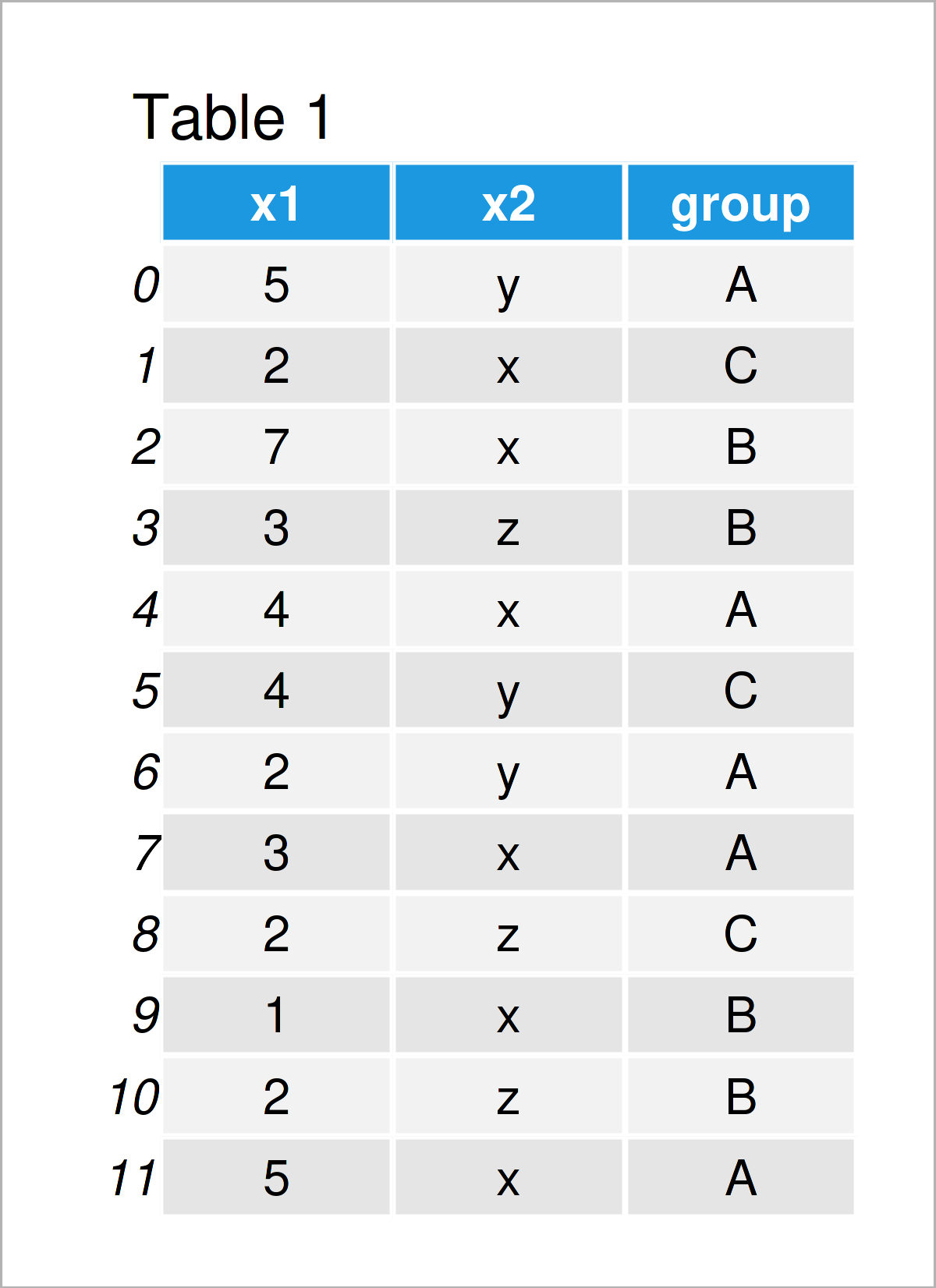# Calculate Mode in Python (4 Examples)

In this article, I’ll explain how to find the mode in the Python programming language.

The page is structured as follows:

You’re here for the answer, so let’s get straight to the Python code:

## Example 1: Mode of List Object

In this example, I’ll demonstrate how to find the mode in a list object in the Python programming language.

Let’s first create an example list:

```my_list = ['x', 'x', 'y', 'x', 'z', 'z']                              # Create example list
print(my_list)                                                        # Print example list
# ['x', 'x', 'y', 'x', 'z', 'z']```

In the next step, we have to import the statistics module:

`import statistics                                                     # Load statistics`

Now, we can apply the mode function provided by the statistics module to calculate the mode of our example list:

```print(statistics.mode(my_list))                                       # Get mode of list
# x```

As you can see, the most common value in our list is the character x.

## Example 2: Mode of One Particular Column in pandas DataFrame

The following Python programming syntax explains how to get the mode of a specific column in a pandas DataFrame.

First, we have to import the pandas library:

`import pandas as pd                                                   # Load pandas library`

Next, we have to create an exemplifying pandas DataFrame:

```data = pd.DataFrame({'x1':[5, 2, 7, 3, 4, 4, 2, 3, 2, 1, 2, 5],       # Create pandas DataFrame
'x2':['y', 'x', 'x', 'z', 'x', 'y', 'y', 'x', 'z', 'x', 'z', 'x'],
'group':['A', 'C', 'B', 'B', 'A', 'C', 'A', 'A', 'C', 'B', 'B', 'A']})
print(data)                                                           # Print pandas DataFrame```By running the previous syntax, we have created Table 1, i.e. a new pandas DataFrame that contains three columns.

Finally, we can apply the mode function to a certain column of our data set (i.e. x1) as shown below:

```print(data['x1'].mode())                                              # Get mode of one column
# 0    2
# dtype: int64```

The previous output shows that the mode of the variable x1 is 2.

## Example 3: Mode of All Columns in pandas DataFrame

The following Python programming syntax shows how to return the most common value in each variable of our pandas DataFrame.

Consider the Python syntax below:

```print(data.mode())                                                    # Get mode of all columns
#    x1 x2 group
# 0   2  x     A```

As you can see, the mode of the column x1 is 2, the mode of the column x2 is x, and the mode of the column group is A.

## Example 4: Mode by Group in pandas DataFrame

In this example, I’ll explain how to GroupBy a pandas DataFrame and select the most common value.

For this task, we have to use the groupby, agg, and value_counts functions as shown in the following Python code:

```print(data.groupby('group').agg(lambda x:x.value_counts().index))  # Get mode by group
#        x1 x2
# group
# A       5  x
# B       1  z
# C       2  y```

The mode values for each column and group are shown in the output above.

## Video, Further Resources & Summary

Would you like to learn more about the calculation of the mode in a list and a pandas DataFrame column? Then you may have a look at the following video that I have published on my YouTube channel. In the video, I explain the content of this article.

Furthermore, you could have a look at the related tutorials on this homepage:

You have learned in this tutorial how to calculate the mode in a list or a pandas DataFrame column in Python programming. Tell me about it in the comments section below, if you have any further questions.

Subscribe to the Statistics Globe Newsletter